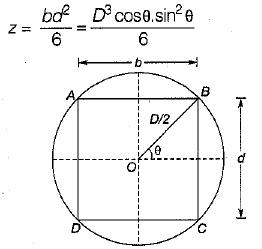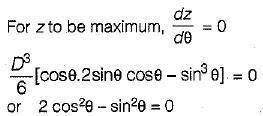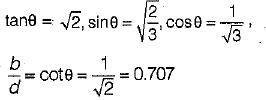Courses

# Test: Properties Of Metals, Simple Stress Strain And Elastic Constants - 1

## 10 Questions MCQ Test Topicwise Question Bank for GATE Civil Engineering | Test: Properties Of Metals, Simple Stress Strain And Elastic Constants - 1

Description
This mock test of Test: Properties Of Metals, Simple Stress Strain And Elastic Constants - 1 for Civil Engineering (CE) helps you for every Civil Engineering (CE) entrance exam. This contains 10 Multiple Choice Questions for Civil Engineering (CE) Test: Properties Of Metals, Simple Stress Strain And Elastic Constants - 1 (mcq) to study with solutions a complete question bank. The solved questions answers in this Test: Properties Of Metals, Simple Stress Strain And Elastic Constants - 1 quiz give you a good mix of easy questions and tough questions. Civil Engineering (CE) students definitely take this Test: Properties Of Metals, Simple Stress Strain And Elastic Constants - 1 exercise for a better result in the exam. You can find other Test: Properties Of Metals, Simple Stress Strain And Elastic Constants - 1 extra questions, long questions & short questions for Civil Engineering (CE) on EduRev as well by searching above.
QUESTION: 1

### A material has identical properties in all directions, it is said to be

Solution:

Isotropic- Identical properties in all directions
Orthotropic- Different properties in all three directions
Homogeneous- A material of uniform composition

QUESTION: 2

### The term nominal stress in stress-strain curve for mild steel implies

Solution: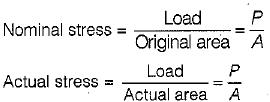Actual area at instant of loading does not remain constant and decreases with increases in elongation actual stress is also called true stress.

QUESTION: 3

### For metallic minerals creep becomes an important consideration at

Solution:

The temperature at which the creep becomes an important consideration is called HOMOLOGOUS TEMPERATURE and this temperature is nearly half of the melting point temperature.

QUESTION: 4

Clapeyron's theorem is associated with the analysis of

Solution:

Clapeyorn’s Theorem of Three Moments express the relation b/w bending moments at three successive supports of a continuous beam, subjected to a loading on two adjacent span with or without settlement of supports.

QUESTION: 5

A rubber band is elongated to double its initial length, its true strain is

Solution: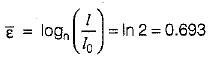QUESTION: 6

A prismatic beam has uniform

Solution: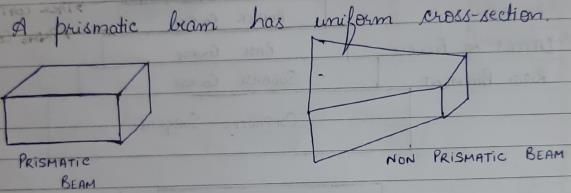QUESTION: 7

In the case of pure bending, the beam will bend into an arc of a

Solution:

In case of pure moment, bending moment at any section will be same. Thus, using double integration method to find deflection we get the equation of parabola.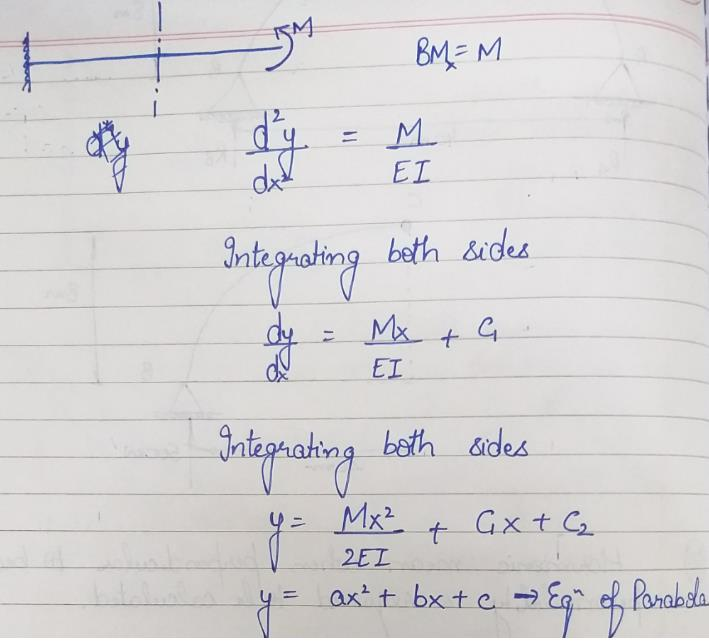QUESTION: 8

If the modulus of elasticity is zero, the material is said to be

Solution:

E =0  Slope of Stress- Strain Curve is straight horizontal line. It means at constant stress, strain keeps increasing which is the characteristic property of perfectly plastic material.

QUESTION: 9

The compressibility of a material is proportional to

Solution: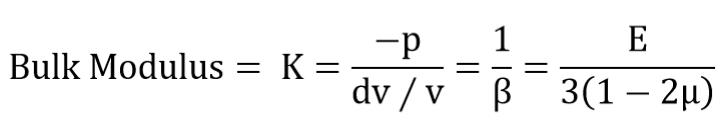Bulk Modulus= K=(-p)/(ⅆv∕v)=1/β=E/3(1-2μ)

β=compressibility is proportional to reciprocal of E.

QUESTION: 10

If a beam with the rectangular cross-section is obtained by cutting from circular log of timber, then for the beam to have strongest section in bending, the ratio of breadth to depth should be

Solution:

AB = b = D cosθ, BC = d = D sinθ